Get instant live expert help with Excel or Google Sheets“My Excelchat expert helped me in less than 20 minutes, saving me what would have been 5 hours of work!”

#### Post your problem and you’ll get expert help in seconds.

Your message must be at least 40 characters
Our professional experts are available now. Your privacy is guaranteed.

# How to Count Characters in Excel

After this tutorial you will be able to count total characters in a cell or range, occurrences of specific character or character combination in a cell or range with both case sensitive and insensitive alternatives. Knowledge gained in this tutorial is a really good base for further creative Excel problem-solving.

## How to Count Characters in Excel

If you want to learn how to count characters in Excel, you need to use function LEN, using formula =LEN(cell) for counting total characters in a cell, or combination of functions SUMPRODUCT and LEN for counting total characters in a range with formula =SUMPRODUCT(LEN(range)). Additionally, counting of a specific character in a cell or range and specific combination of characters in a cell or a range will be explained in detail.

1. Count Total Characters in a Cell
2. Count Total Characters in a Range
3. Count Specific Character in a Cell
4. Count Specific Character in a Range
5. Count Specific Combination of Characters in a Cell or Range

### Count Total Characters in a Cell

Total characters in a cell can be easily found using Excel function LEN. This function has only one argument, cell reference or text, where total character number would like to be counted:

`=LEN(text)`

`=LEN(B3)`

This function counts total characters in a cell, including spaces, punctuation marks, symbols, despite how many times they occur in a string.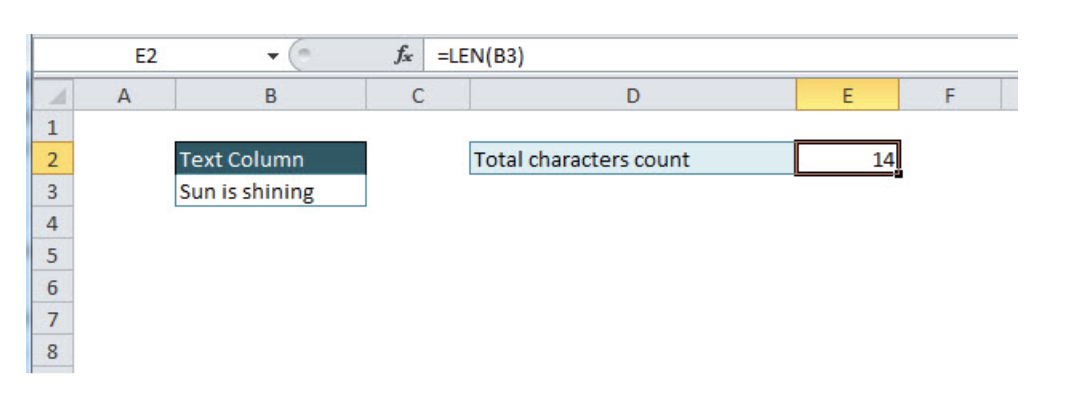If we want to count total characters in a cell, excluding spaces, the combination of formulas LEN and SUBSTITUTE will be required. Let’s take a look in the example below, the formula that excludes spaces looks like: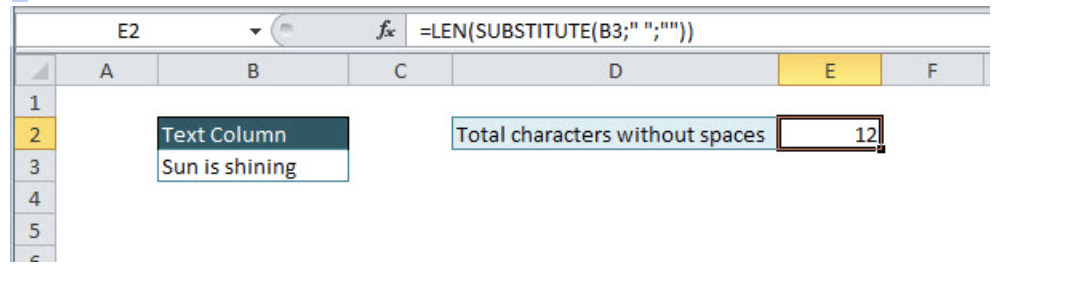`=LEN(SUBSTITUTE(B3;" ";""))`

The SUBSTITUTE function changes in a defined cell one character/text with another character/text. If we want to exclude spaces, using this function, they will be eliminated, replacing all occurrences of spaces with an empty string. After SUBSTITUTE, function text in a cell would look like:

=LEN(“Sunisshining”)

After that, it is easy to count total characters with LEN function, with the final result of 12 characters.

### Count Total Characters in a Range

For counting total character number in a defined range, the combination of two functions is needed, SUMPRODUCT and LEN. SUMPRODUCT function usage is elegant solution whenever we are dealing with multiple cells or arrays. Take a look in the example below and a combination of the formulas:

`=SUMPRODUCT(LEN(range))`

`=SUMPRODUCT(LEN(B3:B6))`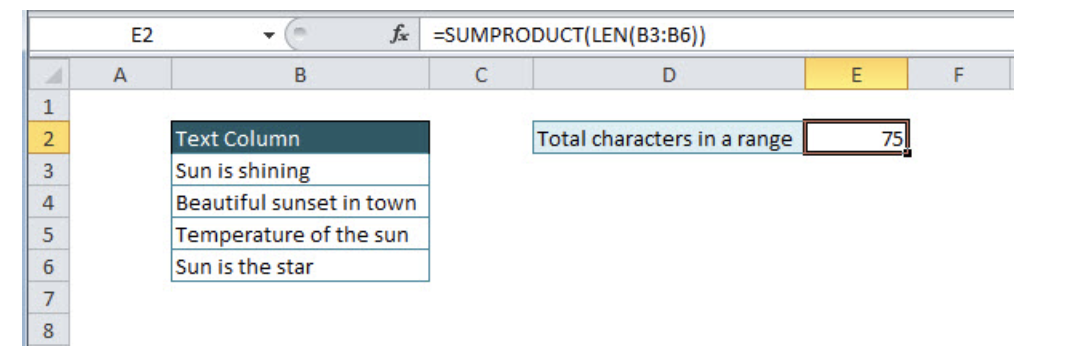LEN function is related to cell, but for cell ranges usage of summed LEN functions (=LEN(B3)+LEN(B4)+LEN(B5)+LEN(B6)) is not the best solution since we could be dealing with huge ranges. Instead of that, SUMPRODUCT function summarizes results of LEN function in the defined range. In formula evaluation, the result will look like:

`=SUMPRODUCT({14;24;22;15})`

Numbers from the array are LEN function results from each cell in the defined range B3:B6, giving final character results of 75.

The alternative solution for dealing with ranges is the usage of SUM and LEN function combination. The syntax is almost the same, with the only difference of curly brackets usage, in order to convert SUM function in array formula (Shortcut for creating an array is CTRL+SHIFT+ENTER). In the formula below is the exact syntax for SUM/LEN function combination:

`={SUM(LEN(B3:B6))}`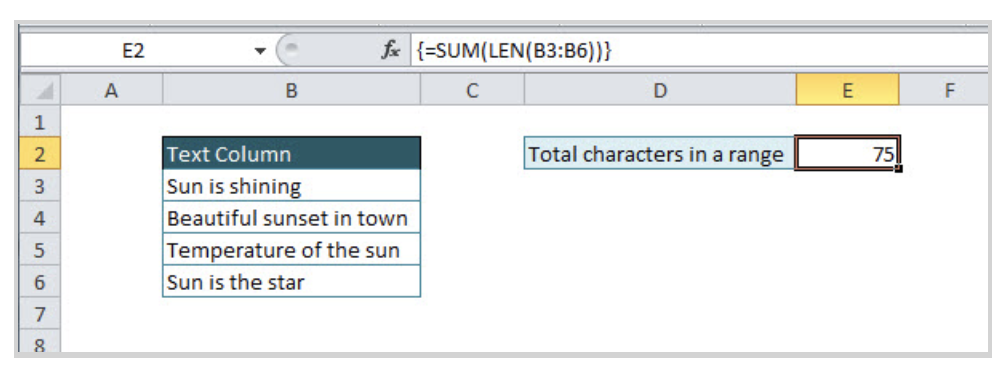### Count Specific Character in a Cell

Besides the total character number, there is also the option in Excel for counting the number of occurrences of specific characters. Let’s go through the example of counting number of single character in a specific cell. For this purpose combination of LEN and SUBSTITUTE function is needed, as we did in the similar example of counting the number of characters in the cell without space. Formula syntax will look like:

`=LEN(cell)-LEN(SUBSTITUTE(cell;character;""))`

In a specific example, if we want to count the number of character s in a defined cell B3, the formula will look like:

`=LEN(B3)-LEN(SUBSTITUTE(B3;"s";""))`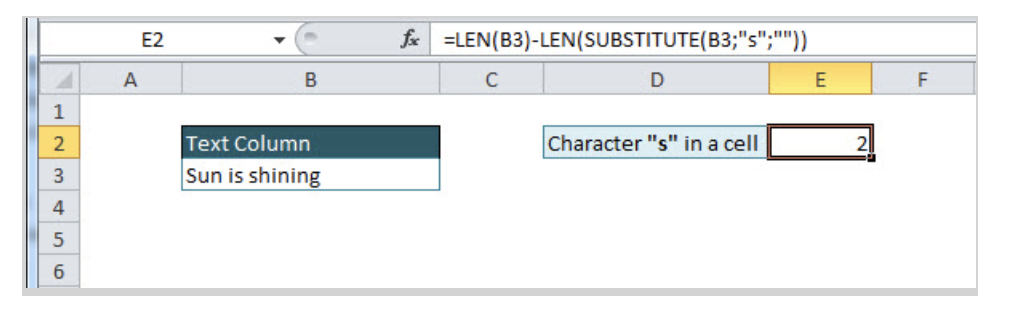Let’s explain briefly logic of the function combination. Total character number in a cell B3 is subtracted with character number in the same cell, but without specific character that we want to count. As mentioned in tutorial point 1, SUBSTITUTE function is used to change a string in a defined cell in form without specific character, replacing that character with an empty string.

`=SUBSTITUTE(cell;"character";"")`

It can be noticed in the final result that function is not counting uppercase characters since LEN function is case sensitive. The solution for counting characters without case sensitive criteria is the usage of UPPER/LOWER function, where all characters will be translated to uppercase/lowercase and function will become case-insensitive.

In the example below, function LOWER is nested into SUBSTITUTE function, changing all string in cell B3 into lowercase, since criteria are defined as lowercase, “s”:

`=LEN(cell)-LEN(SUBSTITUTE(LOWER(cell);"lowercase character";""))`

`=LEN(B3)-LEN(SUBSTITUTE(LOWER(B3);"s";""))`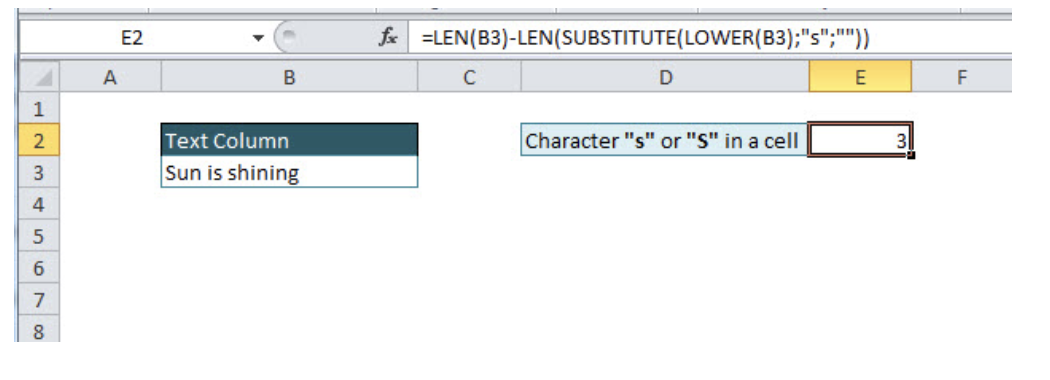Another solution for making non-case sensitive function is the usage of double nested SUBSTITUTE function in combination with LEN function. In the further example, counting character will be in a specific cell, because sometimes it is not practical to write each time to count a character in the formula, especially if you are dealing with complex ranges and formulas.

Formula with double nested SUBSTITUTE function:

`=LEN(cell)-LEN(SUBSTITUTE(SUBSTITUTE(cell;LOWER(character);"");UPPER(character);""))`

`=LEN(B3)-LEN(SUBSTITUTE(SUBSTITUTE(B3;LOWER(B6);"");UPPER(B6);""))`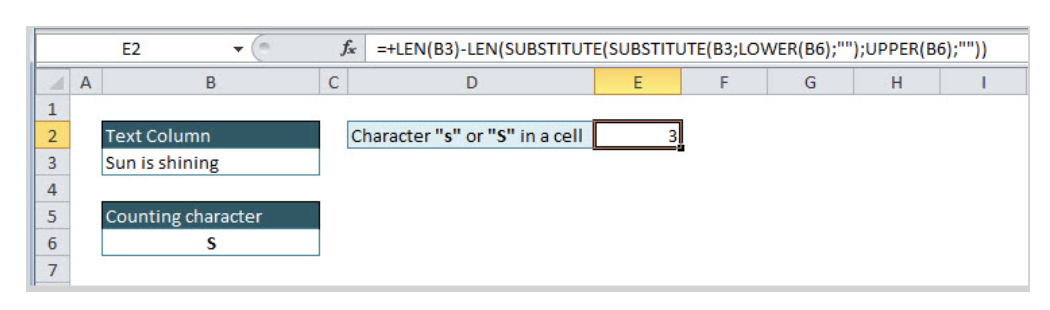The formula might be looking complex, but everything will be clear after explanation. Let’s evaluate formula step by step.

First, we want to eliminate the lowercase counting character from the text:

`=SUBSTITUTE(B3;LOWER(B6);"")`

`=SUBSTITUTE(“Sun is shining ”;LOWER(“S”);"")`

Function LOWER is put in order to translate counting character into lowercase, and then the +SUBSTITUTE function replaces lowercase character into an empty string. After this step formula result look like: “Sun i hining”.

In the next formula step, the goal is to eliminate the uppercase counting character from the defined text/cell. This is solved by nested SUBSTITUTE function:

`=SUBSTITUTE(SUBSTITUTE(B3;LOWER(B6);"");UPPER(B6);"")`

We have already explained inside SUBSTITUTE function result “Sun i hining”, and will put it in the function below to make the situation clearer. In first SUBSTITUTE function lowercase character has been replaced with an empty string, and in the second SUBSTITUTE function uppercase counting character is replaced with an empty string, resulting in the text without counting characters “s” and “S”: “un i hining”.

`=SUBSTITUTE(“Sun i hining”;UPPER(“S”);"")`

LEN function then just counts the number of characters from a modified text:

`=LEN(SUBSTITUTE(SUBSTITUTE(B3;LOWER(B6);"");UPPER(B6);""))`

`=LEN(“un i hining”)`

In the final step, evaluated formula result, text without “s” and “S”, is subtracted by the total number of characters in a defined cell:

`=LEN(B3)-LEN(SUBSTITUTE(SUBSTITUTE(B3;LOWER(B6);"");UPPER(B6);""))`

`=LEN(“Sun is shining”) - LEN(“un i hining”)`

### Count Specific Character in a Range

Whenever we are dealing with cell ranges and arrays, SUMPRODUCT function is needed in combination with other functions. For counting the specific character in a range, for case, sensitive counting, the combination of three functions is used: SUMPRODUCT, LEN, and SUBSTITUTE. For case-insensitive counting, additional formulas should be added: UPPER/LOWER.

First, we will explain counting the number of occurrence of character “S” in a defined range (case insensitive version). A formula is almost similar to formula explaining counting characters. The difference is coming from LEN function nested in SUMPRODUCT function and in counting area, instead of the cell is defined range of cells:

`=SUMPRODUCT(LEN(B3:B6))-SUMPRODUCT(LEN(SUBSTITUTE(B3:B6;B9;"")))`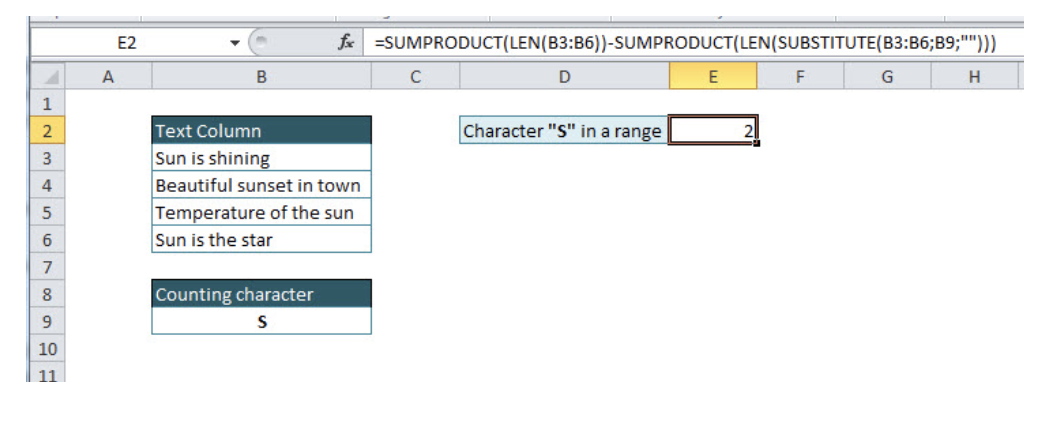For case-insensitive character counting in a defined range, we can use the detailed explained function for case-insensitive character counting in a specific cell in tutorial point 3, with two changes: nesting LEN function in SUMPRODUCT function, and replacing the cell with cell range:

`=SUMPRODUCT(LEN(B3:B6))-SUMPRODUCT(LEN(SUBSTITUTE(SUBSTITUTE(B3:B6;LOWER(B9);"");UPPER(B9);"")))`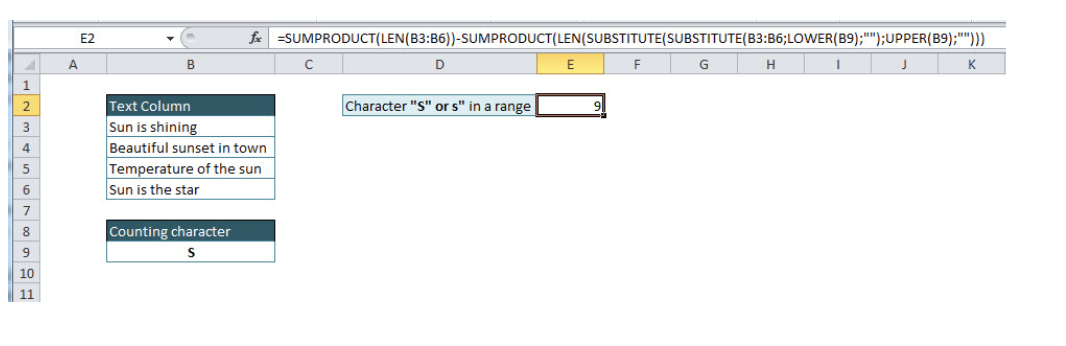Remember, whenever you are dealing with cell ranges, you will have to use SUMPRODUCT function. For case-sensitive character counting in range combination of functions is needed: SUMPRODUCT, LEN and SUBSTITUTE, and for case-insensitive counting: SUMPRODUCT, LEN, SUBSTITUTE, and UPPER/LOWER functions.

### Count Specific Combination of Characters in a Cell or Range

There is also the possibility to count specific character combination in a defined cell or range. In previous tutorial points, we covered single character counting in a cell or range with case sensitive/insensitive alternatives. The formula for counting the combination of characters is the same, only we have to divide it with the number of characters in the character combination.

Let’s take a look in the example below, for counting specific combination of characters in a cell (case insensitive version):

`=(LEN(B3)-LEN(SUBSTITUTE(SUBSTITUTE(B3;LOWER(B6);"");UPPER(B6);"")))/LEN(B6)`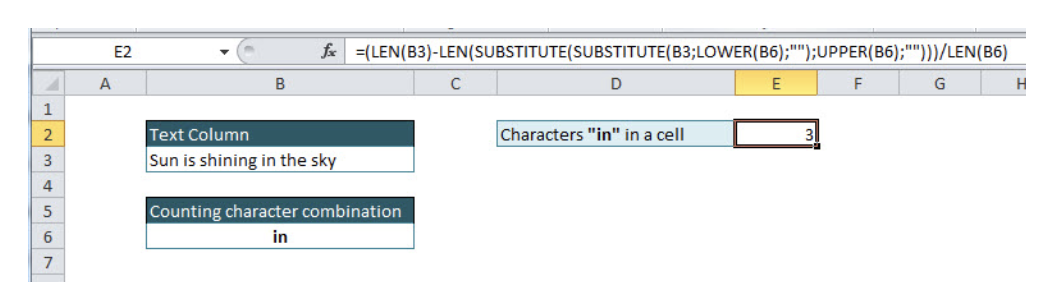Logic is the same as for counting single character, only we had to divide regular formula with the number of specific characters that we are counting, easily using the formula: LEN(“in”). Without dividing formula with LEN(“in”), the result would be multiplied with the number of characters in character combination (in our example with 2, since “in” has two characters)

For counting specific combination of characters in a cell (case sensitive version) formula will look like:

`=(LEN(B3)-LEN(SUBSTITUTE(B3;B6;"")))/LEN(B6)`

Counting combination of characters in a range has the same logic as counting single character, and for the case, sensitive version formula will look like:

`=(SUMPRODUCT(LEN(B3:B6))-SUMPRODUCT(LEN(SUBSTITUTE(SUBSTITUTE(B3:B6;LOWER(B9);"");UPPER(B9);""))))/LEN(B9)`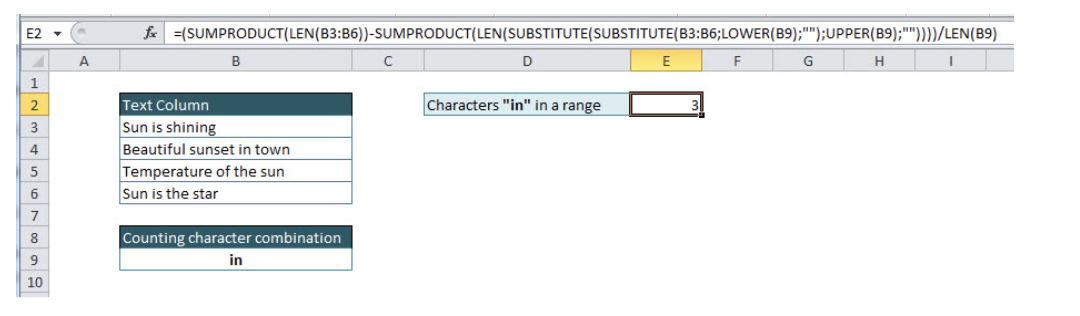Regular formula explained in tutorials topic 4, is divided with the number of specific characters that we are counting, using function LEN.

If we want the case-insensitive version, then formula syntax will look like:

`=(SUMPRODUCT(LEN(B3:B6))-SUMPRODUCT(LEN(SUBSTITUTE(B3:B6;B9;""))))/LEN(B9)`

Still need some help with Excel formatting or have other questions about Excel? Connect with a live Excel expert here for some 1 on 1 help. Your first session is always free.

### Did this post not answer your question? Get a solution from connecting with the expert.Another blog reader asked this question today on Excelchat:
Related blogs
Solution examplesHi - I need a formula that can look at a Member Number (Column A) and report back if location (Column B) matches 1 of 6 locations, then looks to the date of occurance (Column N) but only adds back 1 value of repeating dates. I have this, but it is not coming back with the right answer... =SUMPRODUCT(('2017'!I\$3:I\$10000=\$A223)*ISNUMBER(SEARCH({"2","6","58","69","76","97"},'2017'!N\$3:N\$10000))) Information is corporate private, so I won't be uploading file.
Solved by S. L. in 60 minsI need a formula to pop at the upper left corner of a spreadsheet. If I enter the month "January," I want the column number sum of January =SUM(AB11:AB75) from another section on the same excel page to pop right below the "January" cell, and not display the formula expression, but see the \$100.
Solved by T. Q. in 40 minsCan't add (SUM) in imported numbers from bank account
Solved by F. C. in 40 minshi, i have this formula =SUMPRODUCT((B4:B464<>"")/COUNTIF(B4:B464,B4:B464&"")), i want to add a subtotal to it. so when I filter the column it also sums up the unique number if enteries i have filtered on.
Solved by F. L. in 60 minsI need a formula to combine D2 to D100 to add together a column of numbers, then take away the same amount on the same row when column E is filled. i.e. column D is a price of an item, so the formula must calculate the total, then when the item is sold an 'a' is marked next to the item in column E, the formula then must deduct this amount from the total
Solved by X. W. in 20 mins## Subscribe to Excelchat.coAnother blog reader asked this question today on Excelchat: